Courses

# Fluid Mechanics - 2

## 20 Questions MCQ Test Mock Test Series for SSC JE Mechanical Engineering | Fluid Mechanics - 2

Description
This mock test of Fluid Mechanics - 2 for SSC helps you for every SSC entrance exam. This contains 20 Multiple Choice Questions for SSC Fluid Mechanics - 2 (mcq) to study with solutions a complete question bank. The solved questions answers in this Fluid Mechanics - 2 quiz give you a good mix of easy questions and tough questions. SSC students definitely take this Fluid Mechanics - 2 exercise for a better result in the exam. You can find other Fluid Mechanics - 2 extra questions, long questions & short questions for SSC on EduRev as well by searching above.
QUESTION: 1

### In a flow field, at the stagnation point _______.

Solution:

A stagnation point is a point in a flow field where the local velocity of the fluid is zero. The Bernoulli equation shows that the static pressure is highest when the velocity is zero and hence static pressure is at its maximum value at stagnation points. This static pressure is called the stagnation pressure.

QUESTION: 2

### The number of π parameters needed to express the function F(A, V, t μ, L) = 0 are

Solution:

Total no of π terms = m - n

Here, m = total parameter = 5

n = fluid property, flow property and geometric property (ν, v, L) = 3

∴ no of π – terms = 5 – 3 = 2

QUESTION: 3

### If the particles of a fluid attain such velocities that vary from point to point in magnitude and direction as well as from instant, the flow is _______.

Solution:

In fluid dynamics, turbulent flow is characterized by the irregular movement of particles of the fluid. In contrast to laminar flow the fluid does not flow in parallel layers, the lateral mixing is very high, and there is a disruption between the layers. In turbulent flow the speed of the fluid at a point is continuously undergoing changes in both magnitude and direction.

QUESTION: 4

The relation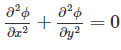for an irrotational flow is known as which one of the following?

Solution:

For three dimensional steady incompressible flow the continuity equation is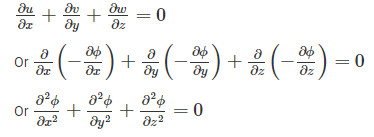Which is nothing but Δ2ϕ  = 0. The “Laplace equation“. Thus any function that satisfied Laplace equation is a possible case of fluid flow.

QUESTION: 5

The flow in a pipe is turbulent when Reynold number is

Solution:

The flow in a circular pipe is

Laminar Flow: Re ≤ 2300

Transitional flow: 2300 ≤ Re ≤ 4000

Turbulent flow: Re ≥ 4000

QUESTION: 6

Kinematic viscosity of water in comparison to mercury is _____.

Solution:

The dynamic viscosity of mercury (1.52 cp) is greater than water (0.894 cp). The kinematic viscosity is the dynamic viscosity divided by the density. Mercury is a lot denser than water, so its kinematic viscosity is lower than the kinematic viscosity of water.

QUESTION: 7

Dynamic viscosity of water in comparison to mercury is

Solution:

Dynamic viscosity of water at 20°C

= 1.005 centipoise

Dynamic viscosity of mercury at 20°C

= 1.6 centipoise

QUESTION: 8

According to newton’s low of viscosity, the shear is directly proportional to:

Solution: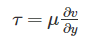Hence shear stress is proportional to velocity gradient.

QUESTION: 9

A fluid of kinematic viscosity 0.5 cm2/sec flows through a 10 cm diameter pipe, The maximum average velocity for laminar flow will be

Solution:

Maximum Reynolds’s number for laminar flow = 2000

We know that, RE=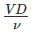∴Maximumvelocity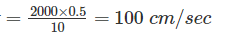QUESTION: 10

Metacentre is the point of intersection of

Solution:

The point ' M ' at which the line of action of the new buoyant force intersects the original vertical through the CG of the body, is called the metacentre. It is a point about which a floating body starts oscillating, when given a small angular displacement.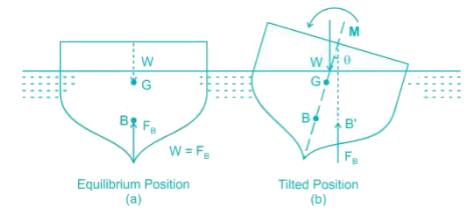GM > 0 (M is above G)                                     Stable equilibrium

GM = 0 (M coinciding with G)                           Neutral equilibrium

GM < 0 (M is below G)                                     Unstable equilibrium

QUESTION: 11

The density of air at 10° C and 1 MPa abs, in SI units is

Solution:

Density (ρ)=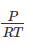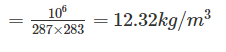QUESTION: 12

A centrifugal pump delivers a liquid when pressure rise in impeller is equal to _______

Solution:

Manometric head is the head against which head is required to be produced by the pump to deliver water to the destination. Manometric head is higher than the sum of suction and delivery heads because it accounts for head losses due to friction.

QUESTION: 13

Separation of flow occurs when pressure gradient

Solution:

Flow separation occurs when the pressure gradient is positive and velocity gradient is negative.

QUESTION: 14

The correct relationship among displacement thickness ‘d’, momentum thickness ‘m’ and energy thickness 'e' is

Solution:

Displacement thickness,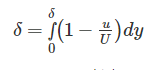Momentum thickness,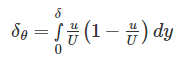Energy thickness,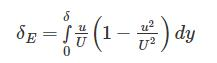δ > δE > δθ

QUESTION: 15

Bernoulli’s equation is applied to

Solution:

Bernoulli's equation states that the summation of pressure head, kinetic head and datum/potential head is constant for steady, incompressible, irrotational and non-viscous flow. In other words an increase in the speed of the fluid occurs simultaneously with a decrease in pressure or a decrease in the fluid's potential energy i.e. the total energy of a flowing system remain constant until external force is applied. So Bernoulli’s equation refers to conservation of energy.

All flow measuring devices like Venturimeter, Orifice meter, Pitot tube meter works on the Bernoulli’s theorem.

QUESTION: 16

Pascal second is the unit of

Solution:

Dynamic viscosity (μ)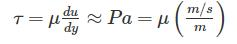μ = Pa – S

QUESTION: 17

Spherical shape of droplets of mercury is due to:

Solution:

Surface tension is responsible for spherical shape of droplets. Surface tension for mercury is 0.485 N/m and that for water is 0.072 N/m.

QUESTION: 18

A stream function is given by (x2 – y2). The potential function of the flow will be

Solution:

Stream function Ψ = x2 – y2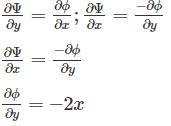Φ = -2xy + C

QUESTION: 19

An accumulator is a device to store

Solution:

An accumulator is a device to store sufficient energy in case of machines which work intermittently to supplement the discharge from the normal source.

QUESTION: 20

In case of power transmission through pipes, maximum efficiency is

Solution:

Efficiency of power transmission is given by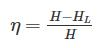For maximum efficiency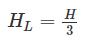We get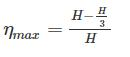ηmax = 66.66%